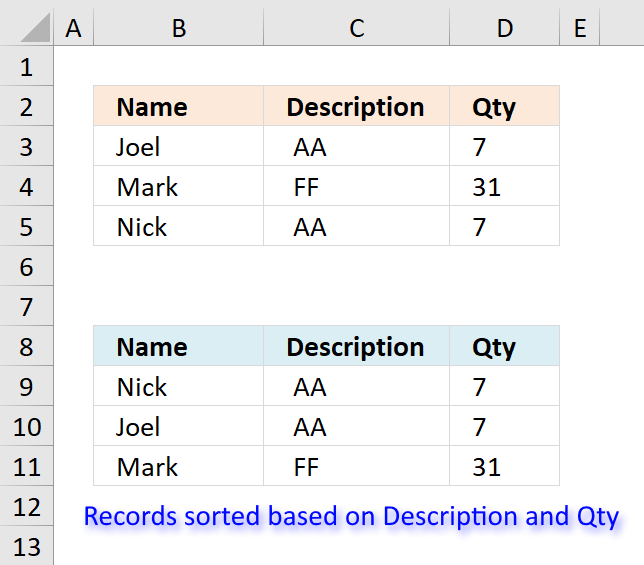Author: Oscar Cronquist Article last updated on January 01, 2019Ralee asks in in this blog post: Sort values in parallel (array formula)

If there is information in adjacent columns, say:

Joel, AA, 7
Mark, FF, 31
Nick, AA, 7

with the possibility of matching sort criteria (columns 2 & 3), how would I display the corresponding data after the sort (sorting only columns 2 & 3) without repeats; that is,

Joel, AA, 7
Nick, AA, 7
Mark, FF, 31

Joel, AA, 7
Joel, AA, 7
Mark, FF, 31

?

Array formula in B9:

=INDEX(\$B\$3:\$B\$5, MATCH(SMALL(COUNTIF(\$C\$3:\$C\$5, "<"&\$C\$3:\$C\$5)+(1/(COUNTIF(\$D\$3:\$D\$5, ">"&\$D\$3:\$D\$5)+1+ROW(\$D\$3:\$D\$5)/65536)), ROWS(\$A\$1:A1)), COUNTIF(\$C\$3:\$C\$5, "<"&\$C\$3:\$C\$5)+(1/(COUNTIF(\$D\$3:\$D\$5, ">"&\$D\$3:\$D\$5)+1+ROW(\$D\$3:\$D\$5)/65536)), 0))

To enter an array formula, type the formula in a cell then press and hold CTRL + SHIFT simultaneously, now press Enter once. Release all keys.

The formula bar now shows the formula with a beginning and ending curly bracket telling you that you entered the formula successfully. Don't enter the curly brackets yourself.

Array formula in C9:

=INDEX(\$C\$3:\$C\$5, MATCH(SMALL(COUNTIF(\$C\$3:\$C\$5, "<"&\$C\$3:\$C\$5), ROWS(\$A\$1:A1)), COUNTIF(\$C\$3:\$C\$5, "<"&\$C\$3:\$C\$5), 0))

Array formula in D9:

=INDEX(\$D\$3:\$D\$5, MATCH(SMALL(COUNTIF(\$C\$3:\$C\$5, "<"&\$C\$3:\$C\$5)+(1/(COUNTIF(\$D\$3:\$D\$5, ">"&\$D\$3:\$D\$5)+1)), ROWS(\$A\$1:A1)), COUNTIF(\$C\$3:\$C\$5, "<"&\$C\$3:\$C\$5)+(1/(COUNTIF(\$D\$3:\$D\$5, ">"&\$D\$3:\$D\$5)+1)), 0))

Explaining array formula in cell B9

Step 1 - Create array containing numbers representing rank order if sorted

The COUNTIF function lets you count values based on a condition. The less than sign lets you compare values based on sort order.

COUNTIF(\$C\$3:\$C\$5, "<"&\$C\$3:\$C\$5)

becomes

COUNTIF({" AA";" FF";" AA"},{"< AA";"< FF";"< AA"})

and returns

{0;2;0}

Step 2 - Create rank order based on second column

Integers represent the sort order of column C and decimal values represent the sort order of column D, combining them creates an array that lets you identify the sort order based on both columns.

1/(COUNTIF(\$D\$3:\$D\$5, ">"&\$D\$3:\$D\$5)+1+ROW(\$D\$3:\$D\$5)/65536)

becomes

1/({0;2;0}+1+ROW(\$D\$3:\$D\$5)/65536)

becomes

1/({0;2;0}+1+{3;4;5}/65536)

becomes

1/({0;2;0}+1+{0.0000457763671875;0.00006103515625;0.0000762939453125})

becomes

1/({1.00004577636718;3.00006103515625;1.00007629394531})

and returns

{0.9999542257282;0.333326551787276;0.999923711875012}

COUNTIF(\$C\$3:\$C\$5,"<"&\$C\$3:\$C\$5)+(1/(COUNTIF(\$D\$3:\$D\$5,">"&\$D\$3:\$D\$5)+1+ROW(\$D\$3:\$D\$5)/65536))

becomes

{0;2;0} + {0.9999542257282;0.333326551787276;0.999923711875012}

and returns

{0.9999542257282;2.33332655178728;0.999923711875012}

Step 4 - Extract k-th smallest value in array

To be able to return a new value in a cell each I use the SMALL function to filter column numbers from smallest to largest.

The ROWS function keeps track of the numbers based on an expanding cell reference. It will expand as the formula is copied to the cells below.

SMALL(COUNTIF(\$C\$3:\$C\$5,"<"&\$C\$3:\$C\$5)+(1/(COUNTIF(\$D\$3:\$D\$5,">"&\$D\$3:\$D\$5)+1+ROW(\$D\$3:\$D\$5)/65536)),ROWS(\$A\$1:A1))

becomes

SMALL({0.9999542257282;2.33332655178728;0.999923711875012},ROWS(\$A\$1:A1))

becomes

SMALL({0.9999542257282;2.33332655178728;0.999923711875012},1)

and returns

0.999923711875012.

Step 5 - Find position of value in array

The MATCH function finds the relative position in a cell range or array.

MATCH(SMALL(COUNTIF(\$C\$3:\$C\$5,"<"&\$C\$3:\$C\$5)+(1/(COUNTIF(\$D\$3:\$D\$5,">"&\$D\$3:\$D\$5)+1+ROW(\$D\$3:\$D\$5)/65536)),ROWS(\$A\$1:A1)),COUNTIF(\$C\$3:\$C\$5,"<"&\$C\$3:\$C\$5)+(1/(COUNTIF(\$D\$3:\$D\$5,">"&\$D\$3:\$D\$5)+1+ROW(\$D\$3:\$D\$5)/65536)),0)

becomes

MATCH(0.999923711875012,COUNTIF(\$C\$3:\$C\$5,"<"&\$C\$3:\$C\$5)+(1/(COUNTIF(\$D\$3:\$D\$5,">"&\$D\$3:\$D\$5)+1+ROW(\$D\$3:\$D\$5)/65536)),0)

becomes

MATCH(0.999923711875012, {0.9999542257282;2.33332655178728;0.999923711875012},0)

and retrurns 3.

Step 6 - Return corresponding value based on position

The INDEX function returns a value based on a cell reference and column/row numbers.

INDEX(\$B\$3:\$B\$5,MATCH(SMALL(COUNTIF(\$C\$3:\$C\$5,"<"&\$C\$3:\$C\$5)+(1/(COUNTIF(\$D\$3:\$D\$5,">"&\$D\$3:\$D\$5)+1+ROW(\$D\$3:\$D\$5)/65536)),ROWS(\$A\$1:A1)),COUNTIF(\$C\$3:\$C\$5,"<"&\$C\$3:\$C\$5)+(1/(COUNTIF(\$D\$3:\$D\$5,">"&\$D\$3:\$D\$5)+1+ROW(\$D\$3:\$D\$5)/65536)),0))

becomes

INDEX(\$B\$3:\$B\$5,3)

and returns "Nick in cell B9.

### Get the Excel fileArray-formula-sorts-columns-in-parallel2.xlsx